## How to get the smallest of zero or more numbers in JavaScript?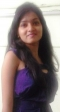Published on 16-Feb-2018 07:12:58
To get the smallest of zero or more number in JavaScript, use the Math.max() method. If no arguments are given, the results is -Infinity.SyntaxThe following in the syntax −Math.min(value1, value2, ... valueN ) ;The parameter value1, value, … value N numbers.ExampleYou can try to run the following code to get ... Read More

## What is different between constant and variable in C++?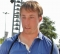Published on 16-Feb-2018 07:13:07
Variable and constant are two commonly used mathematical concepts. Simply put, a variable is a value that is changing or that have the ability to change. A constant is a value which remains unchanged.For example, if you have a program that has a list of 10 radii and you want ... Read More

## How to get the largest of zero or more numbers in JavaScript?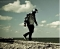Published on 16-Feb-2018 07:11:56
To get the largest of zero or more number in JavaScript, use the Math.max() method. If no arguments are given, the results are -Infinity.SyntaxThe following in the syntax −Math.max(value1, value2, ... valueN ) ;The parameter value1, value, … value N numbers.ExampleYou can try to run the following code to get ... Read More

## How to get the natural logarithm (base E) of a number in JavaScript?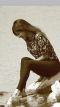Published on 16-Feb-2018 07:09:26
To return the natural logarithm of a number, use the JavaScript Math.log() method. If the value of a number is negative, the return value is always NaN. ExampleYou can try to run the following code to get the natural logarithm of a number −<html>    <head>       <title>JavaScript Math ... Read More

## What are different types of constants in C++?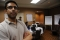Published on 16-Feb-2018 07:07:02
There are no types of constants in C++. It's just that you can declare any data type in C++ to be a constant. If a variable is declared as constant using the const keyword, you cannot reassign its value. Example#include<iostream> using namespace std; int main() {    const int i = ... Read More

## How to define constants in C++?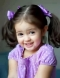Published on 16-Feb-2018 07:06:58
You can define constants in C++ by adding the const qualifier before the declaration of the variable. Example#include<iostream> using namespace std; int main() {    const int x = 9;    x = 0;    return 0; }This will define the constant variable x. But it will throw an error ... Read More

## How to get the largest integer less than or equal to a number in JavaScript?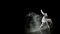Published on 16-Feb-2018 07:04:43
To get the largest integer less than or equal to a number, use the Math.floor() method in JavaScript.ExampleYou can try to run the following code to get the largest integer less than or equal to a number −<html>    <head>       <title>JavaScript Math floor() Method</title>    </head>   ... Read More

## How can I specify the base for Math.log() in JavaScript?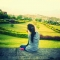Published on 16-Feb-2018 07:01:34
To specify the base for Math.log(), change the base by dividing by log(10).ExampleYou can try to run the following code to implement adding a base for Math.log() −<html>    <head>       <title>JavaScript Math log() Method</title>    </head>    <body>       <script>          var ... Read More

## What are constants in C++?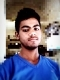Published on 16-Feb-2018 07:02:17
A constant in C++ is a variable/named value that you just want to assign a value to it once and never let it change again in the execution of that program. For example, if you're writing an application that uses the value of pi in a variable PI. You would ... Read More

## How to calculate the ln of a given number in JavaScript?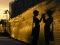Published on 16-Feb-2018 06:59:37
To calculate the ln of a given number, use the Math.log() method in JavaScript. This method returns the natural logarithm (base E) of a number. If the value of a number is negative, the return value is always NaN.ExampleYou can try to run the following code to get the ln ... Read More